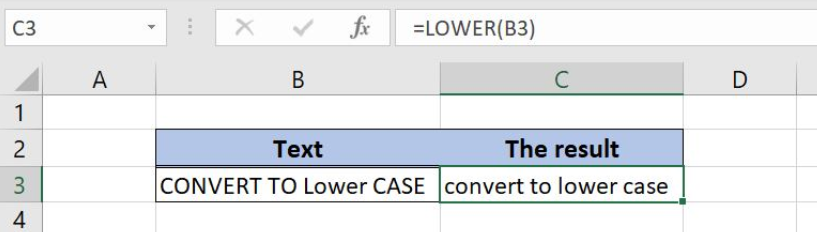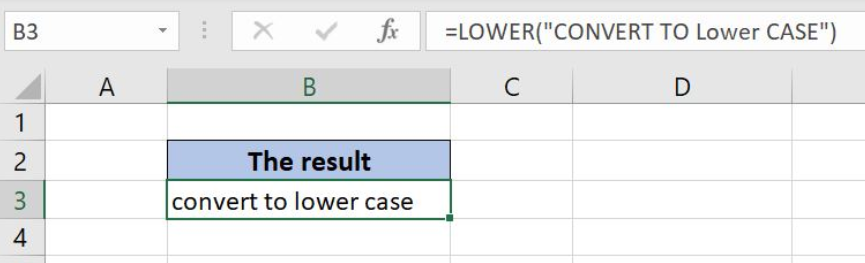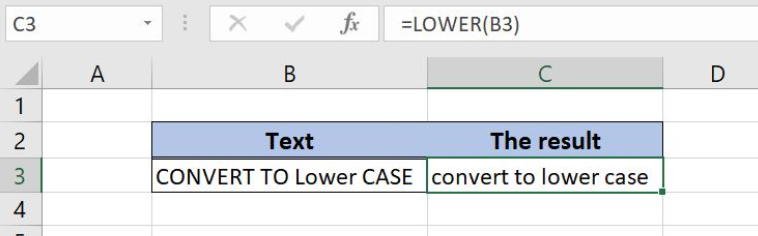Get instant live expert help with Excel or Google Sheets“My Excelchat expert helped me in less than 20 minutes, saving me what would have been 5 hours of work!”

#### Post your problem and you’ll get Expert help in seconds.

Your message must be at least 40 characters
Our professional Expert are available now. Your privacy is guaranteed.

# Excel LOWER Function

Excel allows a user to convert all letters of a string to lower-case, by using the LOWER function. This step by step tutorial will assist all levels of Excel users in converting text to lower-case.Figure 1. The result of the LOWER function

## Syntax of the LOWER Formula

The generic formula for the LOWER function is:

`=LOWER(text)`

The parameter of the LOWER function is:

• text – a text which we want to convert to lower-case.

## Convert the Text to Lower-case Using the LOWER

In our example, we want to convert the text to lower-case. The text is “CONVERT TO Lower case”.

The formula looks like:

`=LOWER("CONVERT TO Lower CASE")`

The parameter text is “CONVERT TO Lower case”.

To apply the IF function, we need to follow these steps:

• Select cell B3 and click on it
• Insert the formula: `=LOWER("CONVERT TO Lower CASE")`
• Press enterFigure 2. Using the LOWER function to convert the text to lower-case

As you can see in Figure 2, all letters of the text are converted to lower-case. Therefore, the result in the cell B3 is “convert to lower case”.

## Using the LOWER Function to Convert Text from the Cell

In this example, we will provide cell reference to the function, instead of text. The example is similar, but now we put the text in the cell B3 and want to get the result in C3.

The formula is:

`=LOWER(B3)`

To apply the IF function, we need to follow these steps:

• Select cell C3 and click on it
• Insert the formula: `=LOWER(B3)`
• Press enterFigure 3. Using the LOWER function to convert the text from the cell

The result is the same as in the previous example. The cell C3 now has the value “convert to lower case”.

Most of the time, the problem you will need to solve will be more complex than a simple application of a formula or function. If you want to save hours of research and frustration, try our live Excelchat service! Our Excel Experts are available 24/7 to answer any Excel question you may have. We guarantee a connection within 30 seconds and a customized solution within 20 minutes.

### Did this post not answer your question? Get a solution from connecting with the expert.Another blog reader asked this question today on Excelchat:
Solution exampleshello i need help creating a formula, if a value does not exsits put 0, if a value exist do nothing
Solved by M. F. in 9 minsCompute the weighted average of students score from a spreadsheet using different weight for each category of marks
Solved by G. E. in 20 minshi there, im making a spread sheet to track progress in a game and would like to create a drop log sheet, i was hoping to use the if function to detect weather a item is logged on a sheet then send the sheet number to a list if so
Solved by K. F. in 60 minsI have a schedule I'm doing with formulas to add workdays (across rows) to a start date column. I would like to write the equation like this: =ifError((workday(b2,1) = cell above, [value of cell above + 1] Help me form this correctly to get the (value of target cell) = (1 more than the value from the cell above) IF the target cell value started equal to the cell above.
Solved by M. J. in 20 minsI need a formula that will subtract the sum of one cell if the sum of another cell is greater than zero, and have it applied to cells across a document that is 30,000 entries and only in certain spots
Solved by F. J. in 20 mins## Subscribe to Excelchat.coAnother blog reader asked this question today on Excelchat: